# Calculated fields sample exercise

Like to learn by doing? Try this sample exercise in creating calculated fields.

## Goals

• Learn how to create a simple arithmetic calculated field.
• Learn how to break more complicated calculations into simpler steps.
• Create a conditional expression to evaluate your data and return different results.

## Scenario

Suppose you manage an online pet supply store. You might track sales data, such product codes (SKU), item names, price, and quantity sold. Here's a simple example:

 SKU Item Price Qty Sold D-001 Healthy Dog Dog Food 79.96 16 B-002 Parrot Perch 74.97 8 B-001 Pretty Bird Bird Seed 31.96 20 C-002 Hungry Kitty Cat Food 29.98 3 D-002 Playful Puppy Toy 17.97 11 C-001 Happy Cat Catnip 14.97 4

Calculated fields in Data Studio can help you manipulate and extend your data. In this exercise, you'll use calculated fields to:

• calculate the total value of each order
• apply a discount to orders over a certain amount
• categorize sales into departments.

### Sample data

To complete this exercise as written, use the sample data below. Otherwise, use your own data (adjusting the field names accordingly). You'll need access to Google Sheets. Alternatively, you could use the File Upload connector to import the data into Data Studio.

Copy and paste this data into a text file:

```SKU,Item,Price,Qty Sold D-001,Healthy Dog Dog Food,79.96,16 B-002,Parrot Perch,74.97,8 B-001,Pretty Bird Bird Seed,31.96,20 C-002,Hungry Kitty Cat Food,29.98,3 D-002,Playful Puppy Toy,17.97,11 C-001,Happy Cat Catnip,14.97,4```

## Instructions

###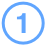Prepare the data

Goal: Create a Google Sheet using the sample data above.
1. Copy the sample data in the green box above.
2. Open a text editor, paste in the sample data, then save the file as `pet-store-data.csv`.

3. Create a new Google Sheet
4. In Sheets, select File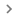Import.
5. Select Upload, then upload the sample data file you created in step 2.
6. Select Replace current sheet.
7. Name your spreadsheet Pet Store.

###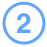Connect to the data

Goal: Create a data source based on the Pet Store spreadsheet.
1. Create a new report in Data Studio.
2. In the bottom right of the new report, click CREATE NEW DATA SOURCE.
3. Select the Google Sheets connector.
4. Select the `Pet Store` spreadsheet you just created.
5. In the upper right, click CONNECT. In a moment, you'll see the columns from the spreadsheet appear as fields in the data source.

###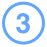Create the subtotal field

Goal: Create a field that calculates the order subtotal.
1. On the top right, click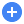ADD A FIELD.
2. In the upper left of the field editor, name this field `Subtotal`.
3. Enter the Formula:  Price * Qty Sold.
The formula editor autocompletes dimension, metric, or function names as you type. You can also drag fields from the Available Fields list on the left
4. On the bottom right, click SAVE.
5. On the left, return to the main data source editor by clicking ALL FIELDS.

###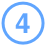Create the discount field

Goal: Create a field that sets the discount rate to apply to orders over a certain value.

Create another calculated field, called `Discount`, that evaluates the Subtotal field: if the amount is more than \$500, set the discount rate to 10%. Otherwise, leave the Subtotal as is.

Hints:

• You can use the CASE statement to perform branching logic in calculated fields.
• In the next step, you'll multiply the Subtotal field by the discount rate. Use .9 to apply a 10% discount.

## Solution

```CASE   WHEN Subtotal > 500 THEN .9   ELSE 1 END```

###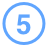Create the Total field

Goal: Create a field that applies the discount rate to the subtotal.

Create another calculated field, called `Total`, that multiples the Subtotal field by the Discount field.

## Solution

`Subtotal * Discount`
Note that you could also have accomplished the same thing by including the subtotal calculation directly:
`(Price * Qty Sold) * Discount`
You could not, however, combine the discount evaluation and the total calculation in one field, as the CASE statement doesn't allow you to do math. For example, the following WILL NOT WORK: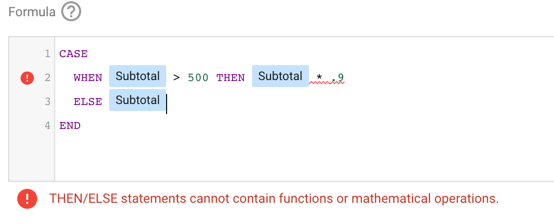Understanding how and when you can accomplish your goal in a single calculated field, and when you'll need to break the calculation into separate steps, will make you more successful with calculated fields.

###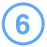Create the Department field

Goal: Create a calculated field that categorizes items sold into departments.

Create a final calculated field, called Department, that parses the SKU field and assigns new values depending on the SKU. You'd like to group dog, cat, and bird related items into separate departments.

Hint: use the `CASE` statement again, this time, with the REGEXP_MATCH function.

## Solution

```CASE   WHEN REGEXP_MATCH(SKU, "^C.*") THEN "Cat"   WHEN REGEXP_MATCH(SKU, "^D.*") THEN "Dog"   WHEN REGEXP_MATCH(SKU, "^B.*") THEN "Bird"   ELSE "Misc" END```

###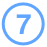See your data in a report

Goal: Visualize your calculated fields.
1. In the upper right, click ADD TO REPORT.
2. In the confirmation dialog, click ADD TO REPORT.
3. Add a table to the report.
4. Add your new calculated fields to see the results.

Was this article helpful?
How can we improve it?